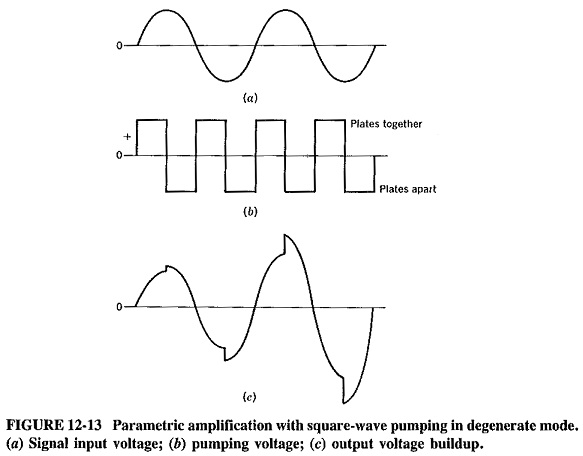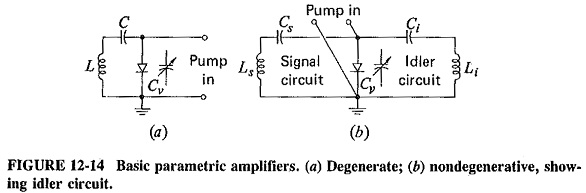## Parametric Amplifier:

The parametric amplifier uses a device whose reactance is varied in such a manner that amplification results. It is low-noise because no resistance need be involved in the amplifying process. A varactor diode is now always used as the active element. Amplification is obtained when the reactance (capacitive here) is varied electronically in some predetermined fashion at some frequency higher than the frequency of the signal being amplified. The name of the amplifier stems from the fact that capacitance is a parameter of a tuned circuit.

### Fundamentals of Parametric Amplifiers:

To understand the operation of one of the forms of the parametric, amplifier, consider an LC circuit oscillating at its natural frequency, If the capacitor plates are physically pulled apart at the instant of time when the voltage between them is at its positive maximum, then work is done on the capacitor since a force must be applied to separate the plates. This work, or energy addition, appears as an increase in the voltage across the capacitor. Since V = q/C and the charge q remains constant, voltage is inversely proportional to capacitance. Since the capacitance has been re­duced by the pulling apart of the plates, voltage across them has increased proportionately. The plates are now returned to their initial separation just as the voltage between them passes through zero, which involves no work. As the voltage passes through the negative maximum, the plates are pushed apart, and voltage increases once again. The process is repeated regularly, so that energy is taken from the “pump” source and added to the signal, at the signal frequency; amplification will take place if an input circuit and a load are connected. In practice, the capacitance is varied electronically (as could be the inductance). Thus the reactance variation can be made at a much faster rate than by mechanical means, and it is also sinusoidal rather than a square wave.

Comparing the principles of the parametric amplifier With those of more conventional amplifiers we see that the basic difference lies in use of a variable reactance (and an ac power-supply), by the former, and a variable resistance (and a dc power supply) by the latter. As an example, in an ordinary transistor amplifier, changes in base current cause changes in collector current when the collector supply voltage is constant; it may be said that the collector resistance is being changed.

The basic parametric amplifier just described requires the capacitance variation to occur at a pump frequency that is exactly twice the resonant frequency of the tuned circuit, and hence twice the signal frequency. It is thus phase-sensitive; this is a property that sometimes limits its usefulness. This mode of operation is called the Degenerate Mode, and it may also be shown that the amplifier is a negative-resistance one.

### Parametric Amplifier Working:

The introduction laid down the basis of parametric amplification, and Figure 12-13 illustrates the process Parametric Amplifier Working. It will be seen that (as outlined) the voltage across the capacitor is increased by pumping at each signal voltage peak. Furthermore, the energy thus given to the circuit is not removed when the plates are restored to their initial position (i.e., when the capacitance of the diode is restored to its original value) because this is done when the voltage across the capacitance is instantaneously zero.The process of signal buildup is shown in Figure 12-13c. Note that it requires more energy in each successive step to increase the voltage across the capacitance, because the peak charge is greater each time. The capacitor voltage would tend to increase indefinitely, except that the driving power is finite. Thus in practice the buildup progresses until the energy added at each peak equals the maximum energy available from the pump source.

### Basic Parametric Amplifiers:

If the pump frequency is other than twice the signal frequency, beating between the two will occur, and a difference signal, called the idler frequency, will appear. The amplitude of this idler signal is equal to the amplitude of the output signal, and its presence is an automatic consequence of using a pump frequency such that fp ≠ 2fs. This means that if the idler signal is suppressed, the amplifier will have no gain.Figure 12-14 shows two simple parametric amplifier circuits. In the basic diagram (Figure 12-14a) degenerate operation takes place, whereas for Figure 12-14b fp ≠ 2fs, and the pumping is called non degenerate. An idler circuit is necessary for amplification to take place, and one is provided. The pump frequency tuned circuit has been left out in each case for the sake of simplicity. Note that nothing prevents us from taking the output at the idler frequency, and in fact there are a number of advantages in doing this.

The non degenerate parametric amplifier, like the degenerate one, produces gain, with the pump source being a net supplier of energy to the tank circuit. This can only be proved mathematically, with the aid of the Manley-Rowe relations. These show that substantial gain is available from this parametric amplifier, in which the pump frequency has no special relationship to the signal frequency (except to be higher, as a general rule). This still holds if sine-wave pumping is used, and it also applies if the output is at the idler frequency.

In the non degenerate parametric amplifier, the energy taken from the pumping source is transformed into added signal-frequency and idler-frequency energy and divides equally ‘between the two tuned circuits. An amplified output may thus be obtained at either frequency, raising the possibility of frequency conversion with gain. In fact, two different types of converters are possible. If the pump frequency is much higher than the signal frequency, then the idler frequency fi, which is given by fi = fp – fs, will be much higher than fs, and the circuit is called an upconversion. If the pump frequency is only slightly higher, fi will be legs than fs, and a down converter, which is rather similar to the mixer in an ordinary radio receiver, will result. These aspects of parametric amplification will be discussed in detail in the next section.

Note finally that there is no compulsion whatever for the pump frequency to be a multiple of the signal frequency in the non degenerate amplifier, in fact, it seldom is a multiple in practice.

Scroll to Top